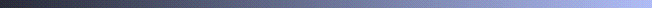# Polynomial-time Techniques for Approximate Timing Analysis of Asynchronous Systems

[Dissertation in compressed PS]Modern high-performance asynchronous circuits are designed with implicit timing assumptions. This facilitates the design of high performance systems with low hardware overhead. However, the correct operation of these systems depends on the validity of the timing assumptions in the actual implementation. This, in turn, depends on the delays of components used in the implementation. To complicate matters, component delays in real systems are often not known precisely owing to statistical variations in manufacturing and operating conditions, data-dependence of delays, etc. Typically, the delay of each component is specified as varying between a best-case and a worst-case value. Consequently, timing analysis techniques for systems with bounded delays are needed.

Unfortunately, several important timing analysis problems for bounded delay systems are computationally intractable. This can pose serious practical problems when analyzing large and complex systems. This research addresses this problem by giving polynomial-time algorithms for approximate timing analysis of asynchronous systems with bounded delays. This contrasts with several previous approaches where exact algorithms with worst-case exponential-time complexity have been used.

The specific problems addressed in this research are:

1. Timing simulation of combinational circuits with bounded gate and wire delays. The goal here is to compute bounds on the signal propagation delays from each primary input to each gate for a given input stimulus. This is important for timing analysis of asynchronous circuits, where the inputs may potentially transition at different times, unlike synchronous circuits. The problem is known to be computationally intractable in circuits with reconvergent fanouts.
2. Time separation of events for bounded-delay systems with min and max timing constraints. Given a timing constraint graph (cyclic in general) modeling the system's temporal behavior, the goal here is to compute time separation bounds between all pairs of events. This is a central problem in the analysis, verification and optimization of asynchronous and concurrent systems. Even for systems modeled by acyclic timing constraint graphs, the problem is NP-complete.
3. Probablistic timing analysis. Component delays in a system may be specified by probability distributions between the best-case and worst-case values. Given such a system, it is often useful to compute the mean, variance and other moments of delays between events for performance analysis purposes. The goal here is to compute the required moments without using Monte Carlo simulation, which can be computationally prohibitive for large systems.